# ML Wiki

## SVM vs Logistic Regression

Recall Logistic Regression:

• the hypothesis is of the form $h_{\theta}(x) = g(\theta^T x) = \cfrac{1}{1 + e^{-\theta^T x}}$
• if $y = 1$ we want $h_{\theta}(x) \approx 1$, or $\theta^T x \gg 0$
• if $y = 0$ we want $h_{\theta}(x) \approx 0$, or $\theta^T x \ll 0$

### Cost Function

Logistic Regression cost function is

• $\text{cost}(h_{\theta}(x), y) = \left\{\begin{array}{l l} -\log(h_{\theta}(x)) & \text{ if } y = 1 \\ - \log(1 - h_{\theta}(x)) & \text{ if } y = 0 \end{array} \right.$
• let's have a look at contribution of each part of the cost function:
• $- \log \cfrac{1}{1 + e^{-z}}$: if $y = 1$, it gives $\theta^T x \gg 0$
• $- \log \left(1 - \cfrac{1}{1 + e^{-z}} \right)$: if $y = 0$, it gives $\theta^T x \ll 0$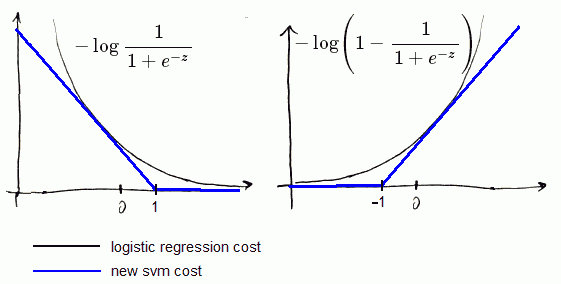Let's change that function onto 2 straight lines:

• one with some slope,
• and second is flat (see the picture)

That gives us

• an approximation of the regression function
• easier optimization

### Objective Function

• $J(\theta) = \cfrac{1}{m} \sum_{i = 1}^{m} \left [ y^{(i)} \cdot \text{cost}_{1}(\theta^T x^{(i)}) + (1 - y^{(i)}) \cdot \text{cost}_{0}(\theta^T x^{(i)}) \right] + \cfrac{\lambda}{2m} \sum_{j = 1}^{n} \theta_j^2$
• where $\text{cost}_{1}(\theta^T x^{(i)})$ and $\text{cost}_{0}(\theta^T x^{(i)})$ are logarithmic cost functions for $y = 1$ and $y = 0$ respectively.
• Let's change them onto svm's cost functions $\text{cost}_{1}(\theta^T x^{(i)})$ and $\text{cost}_{0}(\theta^T x^{(i)})$
• Now, to transform it to svm's objective function:
• we multiply it by $m$,
• divide by $\lambda$,
• and let $C = \cfrac{1}{\lambda}$

Finally we have:

• $J(\theta) = C \cdot \sum_{i = 1}^{m} \left [ y^{(i)} \cdot \text{cost}_{1}(\theta^T x^{(i)}) + (1 - y^{(i)}) \cdot \text{cost}_{0}(\theta^T x^{(i)}) \right] + \cfrac{1}{2} \sum_{j = 1}^{n} \theta_j^2$
• This is just different convention and it doesn't change the value of $\theta$ that minimizes this function

### Hypothesis

The final difference is that

$h_{\theta}(x) = \left\{ \begin{array}{l l} 1 & \text{ if } \theta^T x \geqslant 0 \\ 0 & \text{ otherwise } \end{array} \right.$

## Large Margin

Here's the SVM cost functions: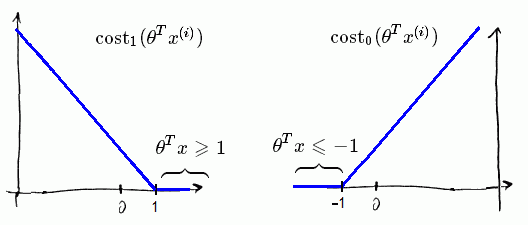Large margin means that

• when $y = 1$ we want $\theta^T x \geqslant 1$, not just $\geqslant 0$
• when $y = 0$ we want $\theta^T x \leqslant -1$, not just $< 0$

That gives larger margin for SVM

### SVM Decision Boundary

• SVM sometimes is referred as Large Margin classifier
• The reason for that is SVM tries to find a decision boundary that has the widest distance (margin from the dataset samples)
•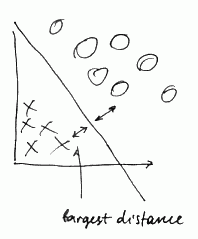• here we see the margin

When $C$ is big, the algorithm becomes sensitive to outliers, decreasing $C$ makes it less sensitive

•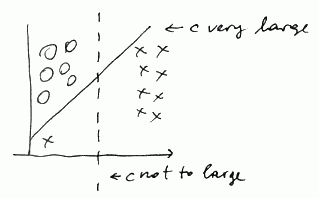### Math Behind It

For SVM, suppose our cost function is

• $J(\theta) = \cfrac{1}{2} \sum_{j = 1}^{n} \theta_j^2$ s.t.
• $\theta^T x^{(i)} \geqslant 1$ if $y^{(i)} = 1$ and
• $\theta^T x^{(i)} \leqslant -1$ if $y^{(i)} = 0$
• For simplification we skip the fist term of our sum and we assume that $\theta_0 = 0$ and number of features $n = 2$
• $\theta^2 = \theta^T \theta$ is an Inner Product
• so we get:
$J(\theta) = \cfrac{1}{2} \sum_{j = 1}^{n} \theta_j^2 = \cfrac{1}{2} (\theta_1^2 + \theta_2^2) = \cfrac{1}{2} \left( \sqrt{\theta_1^2 + \theta_2^2} \right)^2 = \cfrac{1}{2} \| \theta \|^2$
• our optimization objective is to minimize the norm of $\theta$!

Next, let's have a look at $\theta^T \cdot x^{(i)}$

• this is Inner Product as well
• let $p^{(i)}$ be projection from $x^{(i)}$ to $\theta$
•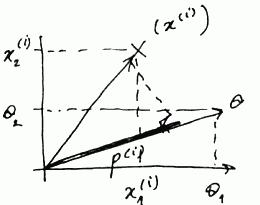• $\theta^T \cdot x^{(i)} = \theta_1 x_1^{(i)} + \theta_2 x_2^{(i)}$
• It means that we can replace the constraints $\theta^T x^{(i)} \geqslant 1$ on $p^{(i)} \| \theta \| \geqslant 1$

Thus we get the following optimization objective:

• $\min_{\theta} \cfrac{1}{2} \sum_{j = 1}^{n} \theta_j^2$, s.t.
• $p^{(i)} \cdot \| \theta \| \geqslant 1$ if $y^{(i)} = 1$
• $p^{(i)} \cdot \| \theta \| \leqslant -1$ if $y^{(i)} = 0$

Suppose we have the following decision boundary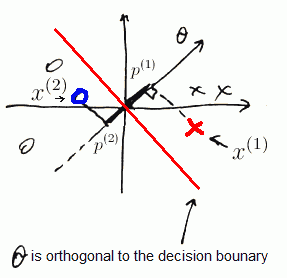But SVM will never give us such line

• both $p^{(1)}$ and $p^{(2)}$ are small numbers (see the picture)
• $\Rightarrow$ $\| \theta \|$ has to be large!

Let's now imagine that SVM chooses

• $OY$ axis as a decision boundary and
• vector $\theta$ is orthogonal - goes along $OX$
•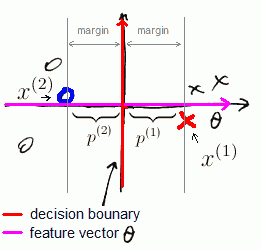Here again we have

• $p^{(1)} > 0$ and $p^{(2)} < 0$, but they are much bigger now
• $\| \theta \|$ doesn't have to be big

That is how SVM gets large margins: because our projections are large.

As a simplification, we assumed $\theta_0 = 0$

• which just means that the boundary always goes through the origin (0, 0)
• if $\theta_0 \neq 0$, it will simply mean that the boundary doesn't pass (0, 0) but otherwise works in exactly same way

## Kernels

Kernels is a technique for using a SVN as a complex, non-linear classifier

Suppose we have the following data

•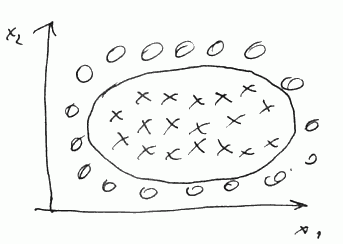• The decision boundary is non linear
• so we predict $y = 1$ if
$\theta_0 + \theta_1 x_1 + \theta_2 x_2 + \theta_3 x_1 x_2 + \theta_4 x_1^2 + + \theta_5 x_2^2 + ... \geqslant 0$

Let's denote each feature as $f$:

• $f_1 = x_1$, $f_2 = x_2$, $f_3 = x_1 x_2$, $f_4 = x_1^2$, $f_5 = x_2^2$
• So we have
$\theta_0 + \theta_1 f_1 + \theta_2 f_2 + \theta_3 f_1 f_2 + \theta_4 f_1^2 + \theta_5 f_2^2 + ... \geqslant 0$

Is there a different / better choice of features $f_1, f_2, f_3, ...$?

### Similarity

Say our $x \in \mathbb{R}^2$

• We may pick up 3 data points, or landmarks: $l^{(1)}, l^{(2)}, l^{(3)}$
•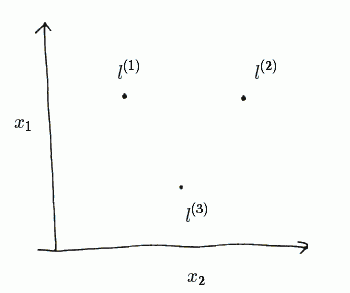• So given a new $x$ we compute new features as proximity to these $l^{(1)}$, $l^{(2)}$ and $l^{(3)}$

We compute $f_1$, $f_2$ and $f_3$ as follows:

• $f_1 = \text{similarity}(x, l^{(1)})$
• $f_2 = \text{similarity}(x, l^{(2)})$
• $f_3 = \text{similarity}(x, l^{(3)})$

These similarity functions are called Kernels

## Gaussian Kernel

As a similarity function we may use a Gaussian Kernel:

• $\text{similarity}(x, l) = \exp \left( \cfrac{\| x - l \|^2 }{2 \cdot \sigma^2} \right)$
• where $\| x - l \|^2 = \sum_{j = 1}^n (x_j - l_j)^2$

Similarity

• Suppose $x$ is close to $l^{(1)}$, i.e. $x \approx l^{(1)}$,
• then the Euclidean distance will be close to 0
• or $f_1 \approx e^0 \approx 1$
• if $x$ is far from $l^{(1)}$ then
• $f_1 \approx \exp \left( \cfrac{(\text{large number})^2}{2 \sigma^2} \right) \to 0$

So each $l^{(1)}, l^{(2)}, l^{(3)}$ defines a feature $f^{(1)}, f^{(2)}, f^{(3)}$ respectively

parameter $\sigma^2$:

• and $\sigma^2$ defines how narrow the gaussian kernels are
• so here's an example with $\sigma^2 = 1$, $\sigma^2 = 0.5$ and $\sigma^2=3$:
•Now given $x$ we can compute all these features

### Example

Suppose we have the following model:

• $\theta_0 + \theta_1 f_1 + \theta_2 f_2 + \theta_3 f_3 \geqslant 0$
• let's say we have the following $\theta$:
$\theta_0 = -0.5, \theta_1 = 1, \theta_2 = 1, \theta_3 = 0$
• which gives us the following contour: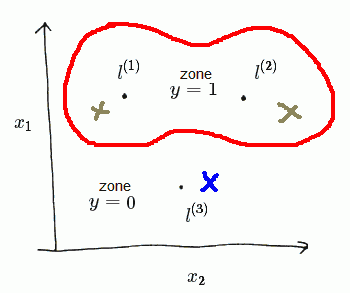Say we have a $x$ near $l^{(1)}$ (green one on the left)

• we have $f^{(1)} \approx 1, f^{(2)} \approx 0, f^{(3)} \approx 0$
• putting it to the model we get
• $\theta_0 + \theta_1 f_1 + \theta_2 f_2 + \theta_3 f_3 \approx -0.5 + 1 = 0.5 \geqslant 0$
• so we predict $y = 1$

Next, say we have a $x$ close to $l^{(3)}$ (blue one on the bottom),

• so $f^{(1)} \approx 0, f^{(2)} \approx 0, f^{(3)} \approx 1$, and we have
• $\theta_0 + \theta_1 f_1 + \theta_2 f_2 + \theta_3 f_3 \approx -0.5 < 0$
• so we predict $y = 0$

Thus in this example

• for points near $l^{(1)}$ and $l^{(2)}$ we end up predicting $y = 1$
• in other cases we predict $y = 0$

### Choosing Landmarks

How to choose the landmarks?

• Suppose we have $m$ training examples $\{(x^{(i)}, y^{(i)})\}$
• Now we just put a landmark at the exactly same locations, i.e. l^{(i)} = x^{(i)}
• And we'll end up with $m$ landmarks $l^{(i)}$

### Usage Notes

• We need to perform Feature Scaling before applying Gaussian Kernel, or one feature may dominate over others.

### Other Kernels

Mercer's Theorem

• Not all similarity functions are valid kernels: they have to satisfy a condition called Mercer's Theorem
• It makes sure they run correctly and don't diverge (to support optimization)

Other kernels:

• No Kernel (or linear kernel)
• predict "$y = 1$" if $\theta^T x \geqslant 0$
• standard linear classifier
• Polynomial Kernel
• String Kernel
• Chi-square Kernel

and so on

## Training

• Given example $x^{(i)}$ we compute
• $f_1^{(i)} = \text{similarity}(x^{(i)}, l^{(1)})$
• $f_2^{(i)} = \text{similarity}(x^{(i)}, l^{(2)})$
• ...
• $f_m^{(i)} = \text{similarity}(x^{(i)}, l^{(m)})$
• And we also add an extra feature $f_0 = 1$ (intercept term)
• That gives us a feature vector $f^{(i)} = [f_0^{(i)}, f_1^{(i)}, ..., f_m^{(i)}]^T \in \mathbb{R}^{m + 1}$
• somewhere in this list we'll have a feature $f_i^{(i)} = \text{similarity}(x^{(i)}, l^{(i)}) = 1$

### Getting $\theta$

• To get $\theta$ we need to train our classifier
• recall that our objective function is
$\min_{\theta} C \cdot \sum_{i = 1}^{m} \left [ y^{(i)} \cdot \text{cost}_{1}(\theta^T x^{(i)}) + (1 - y^{(i)}) \cdot \text{cost}_{0}(\theta^T x^{(i)}) \right] + \cfrac{1}{2} \sum_{j = 1}^{n} \theta_j^2$
• with Kernels, we now have $n = m + 1$ features

Let's take a closer look at the second term

• as we know, this is an Inner Product:
• $\sum_{j = 1}^{n} \theta_j^2 = \| \theta \|^2 = \theta^T \theta$
• In reality, for SVM implementation a re-scaled version is often used:
• $\theta^T \cdot M \cdot \theta$
• which is a numerical optimization trick

### Hypothesis

Given $x$,

• compute features $f \in \mathbb{R}^{m + 1}$
• and predict $y = 1$ if $\theta^T f \geqslant 0$
• ($\theta$ also $\in \mathbb{R}^{m + 1}$)

### SVM parameters

SVM has two parameters

• $C$ (which is equivalent to $\cfrac{1}{\lambda}$ where $\lambda$ is a Regularization term)
• large $C$: lower bias, higher variance (same as small $\lambda$): prone to overfitting
• small $C$: higher bias, lower variance (same as big $\lambda$): prone to underfitting
• $\sigma^2$ for Gaussian Kernels
• large $\sigma^2$: features $f_i$ vary more smoothly, results in higher bias, lower variance
• small $\sigma^2$: features $f_i$ vary less smoothly, results in lower bias, higher variance

### Multi-Class Classification

• Train $K$ SVMs, each to distinguish $y = i$ from the rest
• Get $\theta^{(1)}, \theta^{(2)}, ..., \theta^{(K)}$
• Pick class $i$ with largest $(\theta^{(i)})^T x$

## SVN vs Other Classifiers

### vs Logistic Regression

Suppose we have

• $n$ features ($x \in \mathbb{R}^{n + 1}$)
• $m$ training examples

if $n$ is large (relative to $m$)

if $n$ is small, $m$ in intermediate

• e.g. $n \in [1, 1000], m \in [10, 10000]$
• Use SVM with Gaussian Kernel

if $n$ is small, m is large

• say $n \in [1, 1000], m = 50000+$
• SVM is too slow for that
• create more features
• then use Logistic Regression or SVM without a kernel

Logistic Regression and SVM without a kernel are pretty similar and have similar performance

### vs Neural Networks

• Neural Networks are likely to work for all these cases, but they are slower to train.
• SVM models always have global optimum, whereas Neural Networks have local optima